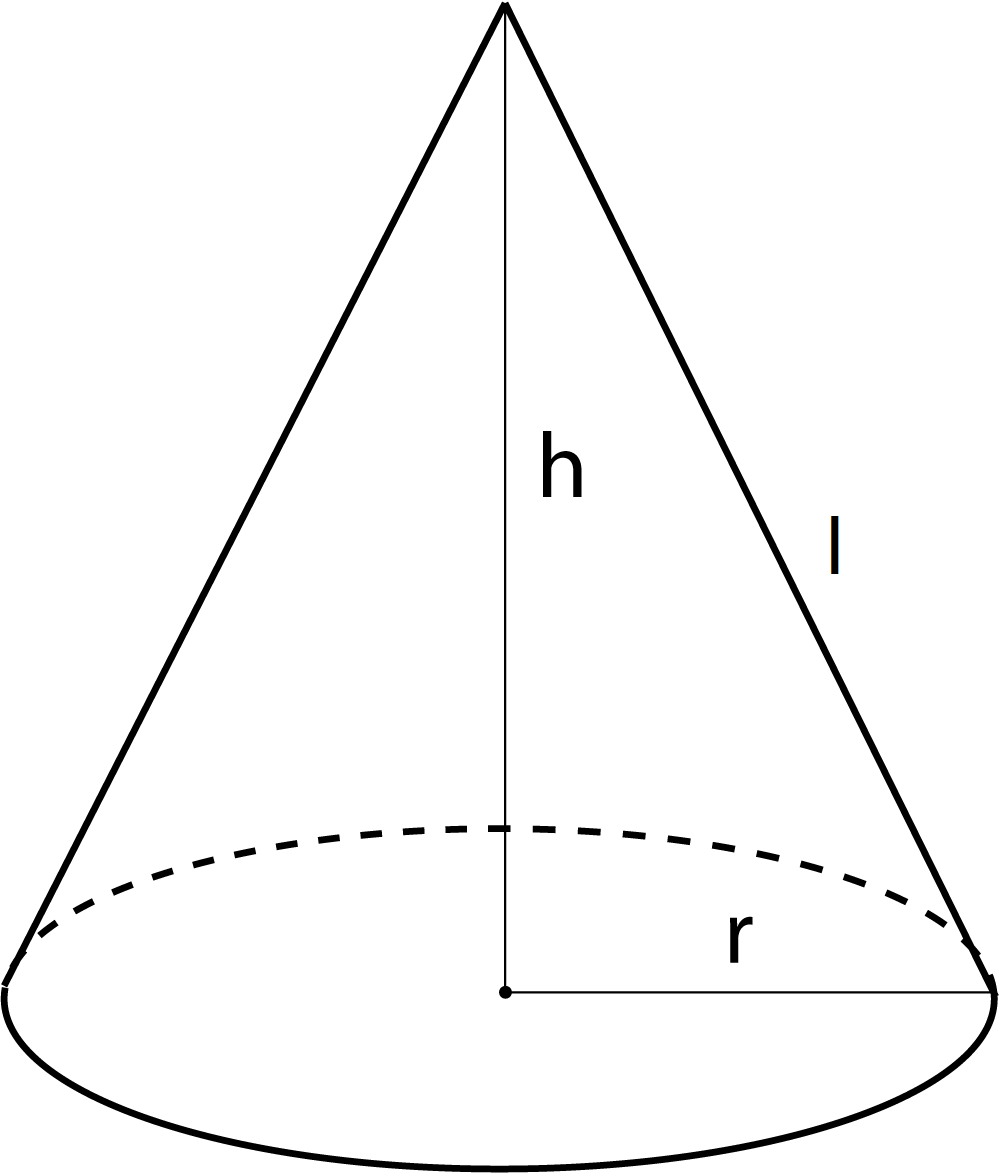Choose language

PL, EN, ES, DE, FR, RU

# Cone calculator - volume, surface area, generatrix, radius of base

The cone calculator will help you calculate the volume of the cone, the total area of the cone, the side area of the cone, the base area of the cone, the generatrix length and the radius of the cone base, and the volume of the sphere described on the cone.

## Slant height (generatrix) of the cone - calculator

$$l = \sqrt {h^2 + r^2}$$

## Lateral surface area of the cone - calculator

$$P_{b} = \pi \cdot r \cdot l$$

## Base area of the cone - calculator

$$P_{p} = \pi \cdot r^2$$

## Surface area of the cone - calculator

$$P_{c} = P_{b} + P_{p}$$ $$P_{c} = \pi r l + \pi r^2 = \pi r(r + l)$$

## Volume of the cone - calculator

$$V = \frac{1}{3}P_{p} \cdot h = \frac{\pi r h}{3}$$

## Volume of the sphere described on the cone - calculator

$$V_{k} = \frac{1}{6} \pi \frac{l^6}{(l^2 - r^2) \sqrt{l^2 - r^2}}$$

Cone - information

Right circular cone is a convex solid formed by the rotation of a right triangle around one of the legs (cathetuses). This cathetus creates the height (h) of the cone, the other leg (cathetus) becomes the radius of the base (r), and the hypotenuse - slant height of the cone (l).Slant height of the cone

Slant height follows from the Pythagorean theorem:
$$l=\sqrt {h^{2}+r^{2}}$$

Lateral surface area of the cone

Lateral surface of the cone, after it is stretched on the plane, forms a circular segment with a radius R = l as the slant height of the cone and the arc length equal to the circumference of the cone base L=2πr
A circular segment with radius R and arc length L has an area: $$P=\frac {1}{2}LR$$ hence $$P_{b}=\frac {1}{2}LR=\frac {1}{2}2\pi rl=\pi rl$$

Base area of the cone

The base of the cone is formed by a circle with a radius r. $$P_{p} = \pi r^2$$

Surface area of the cone

$$P_{c} = P_{b} + P_{p}$$ hence $$P_{c} = \pi r l + \pi r^2 = \pi r(r + l)$$

Volume of the cone

$$V = \frac{1}{3}P_{p}h = \frac{\pi r^2 h}{3}$$

Volume of the sphere described on the cone

$$V_{k} = \frac{1}{6} \pi \frac{l^6}{(l^2 - r^2) \sqrt{l^2 - r^2}}$$

## Users of this calculator also used

### Rail Fence, Zig-Zag - encoder / decoder

Rail Fence, Zig-Zag cipher online encoder and decoder. Encrypt and decrypt any cipher created in a Rail Fence, Zig-Zag cipher.

### Brinell hardness scale

With this calculator you can calculate the HB hardness or the hardness of metals based on the Brinell hardness test.

### Kinetic energy calculator

With this calculator you can quickly calculate the kinetic energy of an object. You will also calculate mass from energy and velocity and speed given kinetic energy and mass.

### Matrix 2x2 calculator

Thanks to the mathematical calculator, you can easily calculate the determinant of the matrix, find the complement matrix, the transposed matrix, and the inverse matrix.

### Dilution of solutions

Using this calculator, you can calculate the total volume of the solution after dilution and the volume of solvent added during dilution.

### Matrix 3x3 calculator

Thanks to the 3x3 matrix math calculator, you can easily calculate the determinant of the matrix 3x3, find the complement 3x3 matrix, transpose 3x3 matrix, inverse 3x3 matrix.

### Vernam cipher - encoder / decoder

Vernam cipher online encoder and decoder. Encrypt and decrypt any cipher created in a Vernam cipher.